Courses

# NCERT Exemplar Solutions: Mensuration Notes | EduRev

## Class 6 : NCERT Exemplar Solutions: Mensuration Notes | EduRev

The document NCERT Exemplar Solutions: Mensuration Notes | EduRev is a part of the Class 6 Course Mathematics (Maths) Class 6.
All you need of Class 6 at this link: Class 6

Exercise Page: 93

In questions 1 to 6, out of the four options only one is correct. Write the correct answer.
Q.1. Following figures are formed by joining six unit squares. Which figure has the smallest perimeter in Fig. 6.4?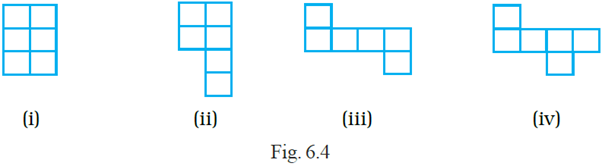Solution:
Figure (i) has the smallest perimeter.
Perimeter of a closed figure is the distance covered in one round along the boundary of the figure.
We know that, perimeter of figure = numbers of sides × length of each sides
Consider the given figures,
(i) figure (i) has 10 units and each side have length 1 cm
So, perimeter = 10 × 1
= 10 cm
(ii) figure (ii) has 12 units and each side have length 1 cm
So, perimeter = 12 × 1
= 12 cm
(iii) figure (iii) has 14 sides and each side have length 1 cm
So, perimeter = 14 × 1
= 14 cm
(iv) figure (iv) has 14 sides and each side have length 1 cm
So, perimeter = 14 × 1
= 14 cm
By comparing all the perimeters of given figures, figure (i) has smallest perimeter.

Q.2. A square shaped park ABCD of side 100m has two equal rectangular flower beds each of size 10m × 5m (Fig. 6.5). Length of the boundary of the remaining park is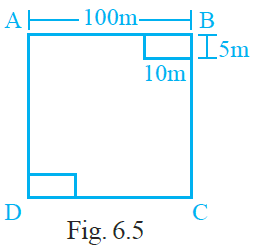(a) 360m
(b) 400m
(c) 340m
(d) 460m
Ans:
(b)
Explanation: We know that, Length of the boundary = Perimeter of boundary
Given, two rectangular flower beds each of size 10m × 5m
So, perimeter of remaining park = 90 + 5 + 10 + 95 + 90 + 5 + 10 + 95
= 400 m

Q.3. The side of a square is 10cm. How many times will the new perimeter become if the side of the square is doubled?
(a) 2 times
(b) 4 times
(c) 6 times
(d) 8 times
Ans: (a)
Explanation: As we know that, perimeter of square = side × 4
= 10 × 4
= 40 cm
Given, side of square = 10 cm
The side of the square is doubled = 10 + 10
= 20 cm
Therefore, the new perimeter becomes 2 times the side of the square is doubled.

Q.4. Length and breadth of a rectangular sheet of paper are 20cm and 10cm, respectively. A rectangular piece is cut from the sheet as shown in Fig. 6.6. Which of the following statements is correct for the remaining sheet?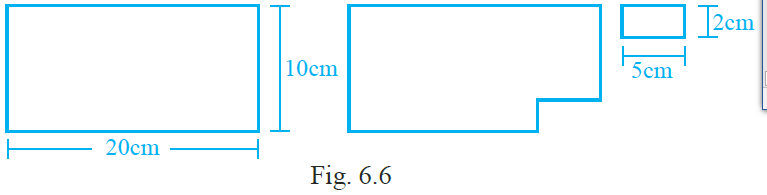(a) Perimeter remains same but area changes.
(b) Area remains the same but perimeter changes.
(c) Both area and perimeter are changing.
(d) Both area and perimeter remain the same.
Ans:
(a)
Explanation:
(A) Perimeter remains same but area changes.
We know that, area of big rectangle = length × breadth
= 10 × 20
= 200 cm2
Area of small rectangle = 5 × 2
= 10 cm2
Perimeter of rectangle = 2(length + breadth)
= 2(20 + 10)
= 2 × 30
= 60 cm
Then, perimeter of new figure = 20 + 8 + 5 + 2 + 15 + 10
= 60
Area of new figure = Area of big rectangle – Area of new figure
= 200 – 10
= 190 cm2
By comparing all the results, Perimeter remains same but area changes.

Q.5. Two regular Hexagons of perimeter 30cm each are joined as shown in Fig. 6.7. The perimeter of the new figure is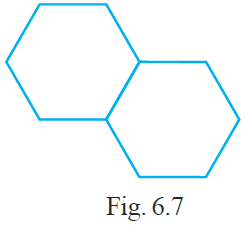(a) 65cm
(b) 60cm
(c) 55cm
(d) 50cm
Ans:
(d)
Explanation:
(D) 50cm
From the question it is given that, perimeter of each hexagon = 30 cm
We know hat, hexagon has 6 sides.
So, length of each side of hexagon = 30/6
= 5 cm
Now, consider two hexagons are joined together.
Then, perimeter of new figure = number of sides × length of each side
= 10 × 5
= 50 cm

Q.6. In Fig. 6.8 which of the following is a regular polygon? All have equal sides except (i)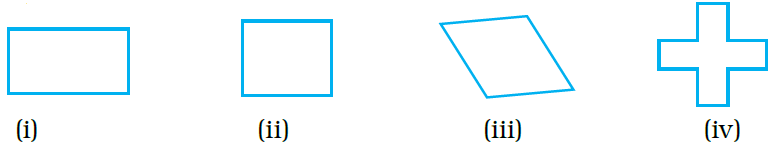(a) (i)
(b) (ii)
(c) (iii)
(d) (iv)
Ans: (b)
Explanation: A closed figure in which all sides and angles are equal is called a regular polygon.

Q.7. Match the shapes (each sides measures 2cm) in column I with the corresponding perimeters in column II: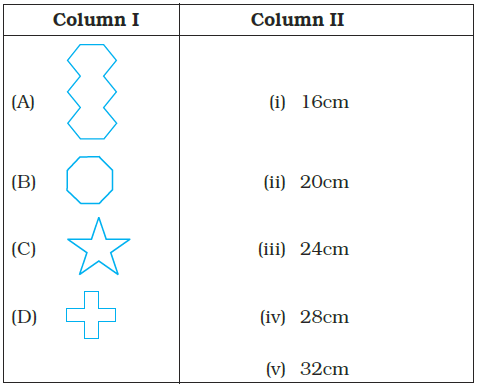Solution:
Given, each side measures 2cm.
Figure (A) has 14 sides.
Perimeter of figure = number of sides × length of each side
= 14 × 2
= 28 cm
Figure (B) has 8 sides.
Perimeter of figure = number of side × length of each side
= 8 × 2
= 16 cm
Figure (C) has 10 sides.
Perimeter of figure = number of sides × length of each side
= 10 × 2
= 20 cm
Figure (D) has 12 sides.
Perimeter of figure = number of sides × length of each side
= 12 × 2
= 24 cm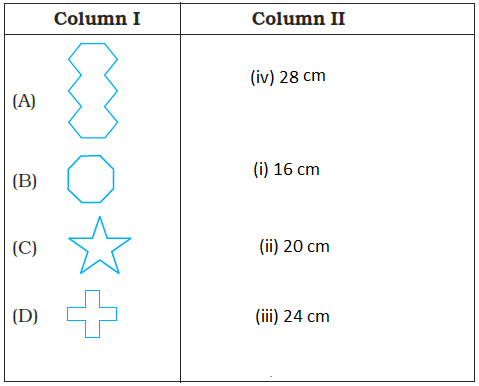Q.8. Match the following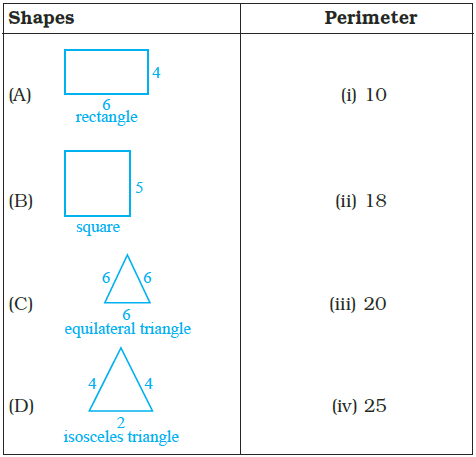Solution:
(A) Perimeter of a rectangle = 2 × (length + breadth)
= 2 × (6 + 4)
= 2 × 10
= 20
(B) Perimeter of a square = 4 × length of its side
= 4 × 5
= 20
(C) Perimeter of an equilateral triangle = 3 × length of a side
= 3 × 6
= 18
(D) perimeter of isosceles triangle = sum of length of all sides
= 4 + 4 + 2
= 10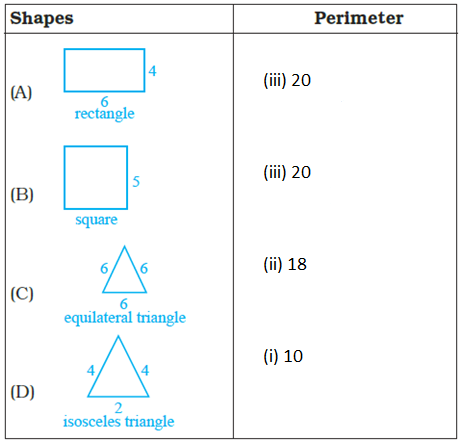In questions 9 to 13, fill in the blanks to make the statements true.
Q.9. Perimeter of the shaded portion in Fig. 6.9 is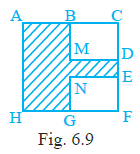AB + _ + _ + _ + _ + _ + _ + HA
Solution: We know that perimeter of a figure = sum of length of all sides
= AB + BM + MD + DE + EN + NG + GH + HA

Q.10. The amount of region enclosed by a plane closed figure is called its _________.
Solution: The amount of region enclosed by a plane closed figure is called its area.

Q.11. Area of a rectangle with length 5cm and breadth 3cm is _________.
Solution: Area of a rectangle with length 5cm and breadth 3cm is 15 cm.
Area of rectangle = length × breadth
= 5 cm × 3 cm
= 15 cm

Q.12. A rectangle and a square have the same perimeter (Fig. 6.10).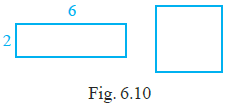(a) The area of the rectangle is _________.
Solution: The area of the rectangle is 12 sq. units.
From figure, length of rectangle is 6 and breadth is 2.
Then, area of rectangle = length × breadth
= 6 × 2
= 12 sq. units
Perimeter of rectangle = 2 × (length + breadth)
= 2 × (6 + 2)
= 2 × 8
= 16 units

(b) The area of the square is _________.
Solution: The area of the square is 16 sq. Units.
From the question it is given that, rectangle and a square have the same perimeter.
So, length of each side of a square = 16/4
= 4 units.
Area of square = side × side
= 4 × 4
= 16 sq. Units

Q.13. (a) 1m = _________ cm.
Solution: 1m = 100 cm.

(b) 1sqcm = _________ cm × 1cm.
Solution: 1sqcm = 1 cm × 1cm.

(c) 1sqm = 1m × _________ m = 100cm × _________ cm.
Solution: 1sqm = 1m × 1 m
= 100cm × 100 cm.

(d) 1sqm = _________ sqcm.
Solution: 1sqm = 10000 sqcm.
We know that, 1 m = 100 cm
Then, 1 sqm = 100 × 100
= 10000sqcm

In questions 14 to 20, state which of the statements are true and which are false.
Q.14. If length of a rectangle is halved and breadth is doubled then the area of the rectangle obtained remains same.
Solution: True.
We know that, area of rectangle = length × breadth
As per the condition given in the question, length of a rectangle is halved = l/2
Then, new area = l/2 × 2b
= l × b.
Therefore, if length of a rectangle is halved and breadth is doubled then the area of the rectangle obtained remains same.

Q.15. Area of a square is doubled if the side of the square is doubled.
Solution: False
We know that, area of square = side × side
As per the condition given in the question, side of the square is doubled = 2 × side
Then, new area = 2side × 2side
= 4 side2
Therefore, if the side of the square is doubled then the area of the square obtained is 4 times the old area.

Q.16. Perimeter of a regular octagon of side 6cm is 36cm.
Solution: False.
Perimeter of regular octagon = number of sides × length of reach sides
= 8 × 6
= 48 cm

Q.17. A farmer who wants to fence his field, must find the perimeter of the field.
Solution: True.

Q.18. An engineer who plans to build a compound wall on all sides of a house must find the area of the compound.
Solution: False.
An engineer who plans to build a compound wall on all sides of a house must find the perimeter of the compound.

Q.19. To find the cost of painting a wall we need to find the perimeter of the wall.
Solution: False.
To find the cost of painting a wall we need to find the area of the wall.

Q.20. To find the cost of a frame of a picture, we need to find the perimeter of the picture.
Solution: True.

Q.21. Four regular hexagons are drawn so as to form the design as shown in Fig. 6.11. If the perimeter of the design is 28cm, find the length of each side of the hexagon.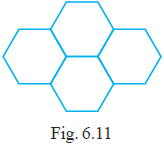Solution:
From the question it is given that, the perimeter of the design is 28cm.
Given design has 14 sides, so length of each side = 28/14
= 2 cm
In the given figure, 4 regular hexagons are joined.
Therefore, the length of each side of the hexagon = 2 cm

Q.22. Perimeter of an isosceles triangle is 50cm. If one of the two equal sides is 18cm, find the third side.
Solution: We know that, isosceles triangle contains two equal sides.
From the question it is given that, one of the two equal sides is 18cm.
And Perimeter of an isosceles triangle is 50cm
We know that, perimeter of isosceles triangle = sum of all sides
Let us assume the third side be x.
Then,
50 = 18 + 18 + x
50 = 36 + x
X = 50 – 36
X = 14 cm
Therefore, the length of third side of an isosceles triangle is 14 cm.

Q.23. Length of a rectangle is three times its breadth. Perimeter of the rectangle is 40cm. Find its length and width.
Solution: From the question it is given that,
Perimeter of rectangle = 40 cm
Length of a rectangle is three times its breadth = 3b
We know that, perimeter of rectangle = 2 × (length + breadth)
40 = 2 × (3b + b)
40 = 2 × 4b
40 = 8b
40/8 = b
b = 5 cm
Therefore, width of rectangle = 5 cm
Length of rectangle = 3b
= 3 × 5
= 15 cm

Q.24. There is a rectangular lawn 10m long and 4m wide in front of Meena’s house (Fig. 6.12). It is fenced along the two smaller sides and one longer side leaving a gap of 1m for the entrance. Find the length of fencing.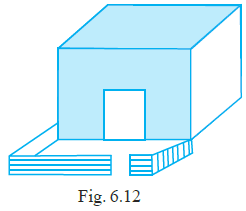Solution:
From the question it is given that,
Length of rectangular lawn = 10m
Width of rectangular lawn = 4m
Then,
Perimeter of fencing = length of fencing
Perimeter of fencing = two smaller sides + one longer side – gap in the longer side
= 4 + 4 + 10 – 1
= 18 – 1
= 17 m
Therefore, length of fencing is 17 m.

25. The region given in Fig. 6.13 is measured by taking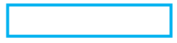as a unit. What is the area of the region?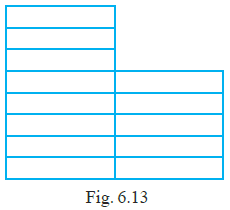Solution:
From the question it is given that, a region measured by taking rectangle is as one unit.
Given figure contain 13 rectangles.
Therefore, area of the region = 13 × 1
= 13 sq. Units

Q.26. Tahir measured the distance around a square field as 200 rods (lathi). Later he found that the length of this rod was 140cm. Find the side of this field in metres.
Solution: From the question it is given that,
Tahir measured the distance around a square field as 200 rods
Length of the rod = 140 cm
So, total distance of square field = 200 × 140
= 28000 cm
Then, length of one side of square field = 28000/4
= 7000 cm
We know that, 1 m = 100 cm
Therefore, side of field = 7000/100
= 70 m

Q.27. The length of a rectangular field is twice its breadth. Jamal jogged around it four times and covered a distance of 6km. What is the length of the field?
Solution: From the question it is given that,
The length of a rectangular field is twice its breadth = 2b
Perimeter of rectangular field = 6km/4
= 1.5 km
We know that, perimeter of rectangle = 2 × (length + breadth)
1.5 = 2 × (2b + b)
1.5 = 2 × 3b
1.5 = 6b
b = 1.5/6
b = 0.25 km
length of rectangle field = 2 × 0.25 = 0.5km
= 0.5 × 1000
= 500 m

Q.28. Three squares are joined together as shown in Fig. 6.14. Their sides are 4cm, 10cm and 3cm. Find the perimeter of the figure.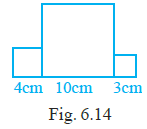Solution: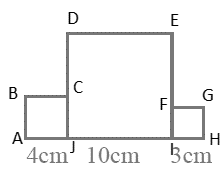We know that, perimeter of figure = sum of all sides
= AB + BC + CD + DE + EF + FG + GH + HI + JI +JA
= 4 + 4 + (10 – 4) + 10 + (10 – 3) + 3 + 3 + 3 + 10 + 4
= 4 + 4 + 6 + 10 + 7 + 3 + 3 + 3 + 10 + 4
= 54 cm

Q.29. In Fig. 6.15 all triangles are equilateral and AB = 8 units. Other triangles have been formed by taking the mid points of the sides. What is the perimeter of the figure?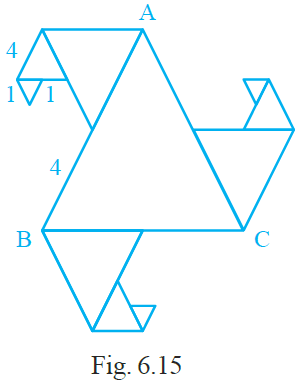Solution:
45 units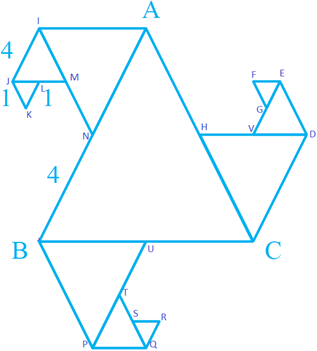Perimeter of given figure = sum of length of each side
= BN + NM + ML + LK + KJ + JI + IA + AH + HV + VG + GF + FE + ED + DC + UC + UT + TS + SR + RQ + PQ + BP
= 4 + 2 + 1 + 1 + 1 + 2 + 4 + 4 + 2 + 1 + 1 + 1 + 2 + 4 + 4 + 2 + 1 + 1 + 1 + 2 + 4
= 45 units

Q.30. Length of a rectangular field is 250m and width is 150m. Anuradha runs around this field 3 times. How far did she run? How many times she should run around the field to cover a distance of 4km?
Solution: From the question it is given that,
Length of a rectangular field is 250m
Width of rectangular field = 150 m
Perimeter of rectangle = 2 × (length + breadth)
= 2 × (250 + 150)
= 2 × 400
= 800 m
Given that, Anuradha runs around this field 3 times = 3 × 800
= 2400 m
We know that, 1km = 1000 m
So, 2400/1000
= 2.4 km i.e. 2 km 400 m
Number of times Anuradha should run around the field to cover a distance of 4km = 4000/800
= 5 times
Therefore, 5 times Anuradha should run around the field to cover a distance of 4km.

Offer running on EduRev: Apply code STAYHOME200 to get INR 200 off on our premium plan EduRev Infinity!

## Mathematics (Maths) Class 6

185 videos|229 docs|43 tests

,

,

,

,

,

,

,

,

,

,

,

,

,

,

,

,

,

,

,

,

,

;